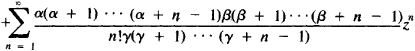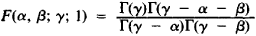# Hypergeometric Series

(redirected from Gauss hypergeometric function)

## hypergeometric series

[‚hī·pər‚jē·ə′me·trik ′sir·ēz]
(mathematics)
A particular infinite series which in certain cases is a solution to the hypergeometric equation, and having the form:
McGraw-Hill Dictionary of Scientific & Technical Terms, 6E, Copyright © 2003 by The McGraw-Hill Companies, Inc.
The following article is from The Great Soviet Encyclopedia (1979). It might be outdated or ideologically biased.

## Hypergeometric Series

a series of the form

F(α, β; γ; z) = 1The hypergeometric series was studied for the first time by L. Euler in 1778. Expansions of many functions into infinite series are special cases of hypergeometric series. For example,The hypergeometric series has meaning if γ is not equal to zero or a negative integer. It converges at |z| < 1. If, in addition, γ - α - β > 0, then the hypergeometric series also converges for z = 1. In this case, Gauss’ formula is valid:where Γ(z) is the gamma function. An analytic function, defined for |z| < 1 by a hypergeometric series is called a hypergeometric function and plays an important role in the theory of differential equations.

References in periodicals archive ?
(2.1) and (2.2)] made use of the extended beta function [B.sub.p]([alpha], [beta]) in (1) to extend the Gauss hypergeometric function and confluent hypergeometric function as follows:
PEREZ SINUSIA, A simplification of Laplace's method: applications to the gamma function and the Gauss hypergeometric function, J.
It has been shown by van der Merwe and Roux  that the above density can be obtained as a limiting case of a density involving the Gauss hypergeometric function of matrix argument.
Let [alpha] > 0, [beta], [eta] [member of] M then the Saigo fractional integral [I.sup.[alpha],[beta],[eta].sub.0,t] (in terms of the Gauss hypergeometric function) of order a for a real-valued continuous function f (t) is defined by (, see also [13, p.
In terms of the Gauss hypergeometric function, the complete elliptic integral of the first kind can be expressed as
Applying the transformation (see [6, [section]2.8]) in terms of the Gauss hypergeometric function
To do this, we consider the Gauss hypergeometric function F (a, b; c; z), which is defined by (see )
The results such as the cumulative distribution function, the mean and the variance of the beta type 3 random variable involve Gauss hypergeometric function which is easily computable using the software Mathematica.
Note that (42) applies also for N = 0, that is, for [epsilon] = 0, for which the Heun function degenerates to the Gauss hypergeometric function u = [sub.2][F.sub.1]([alpha], [beta]; [gamma]; z) provided q = 0.
where F(a,b;c;x) denotes the Gauss hypergeometric function, and the parameters involved are complex numbers.

Site: Follow: Share:
Open / Close